## Tamilnadu Samacheer Kalvi 9th Maths Solutions Chapter 5 Coordinate Geometry Ex 5.1

Question 1.
Plot the following points in the coordinate system and identify the quadrants P(-7, 6), Q(7, -2), R(-6, -7), S(3, 5) and T(3, 9)
Solution: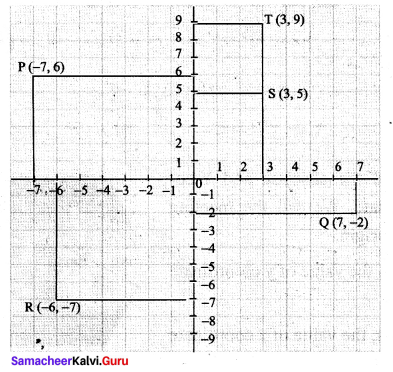(i) P(-7, 6) = II Quadrant
(ii) Q(7, -2) = IV Quadrant
(iii) R(-6, -7) = III Quadrant
(iv) S(3, 5) = I Quadrant
(v) T(3, 9) = I QuadrantQuestion 2.
Write down the abscissa and ordinate of the following
(i) P
(ii) Q
(iii) R
(iv) S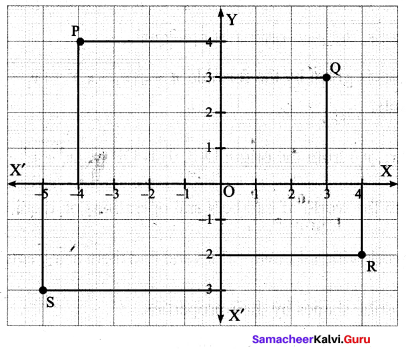Solution:
(i) P(-4, 4)
Abscissa is -4
Ordinate is 4.

(ii) Q(3, 3)
Abscissa is 3
Ordinate is 3

(iii) R(4, -2)
Abscissa is 4
Ordinate is -2

(iv) S(-5, -3)
Abscissa is -5
Ordinate is -3Question 3.
Plot the following points in the coordinate plane and join them. What is your conclusion about the resulting figure?
(i) (-5, 3) (-1, 3) (0, 3) (5, 3)
(ii) (0, -4) (0, -2) (0, 4) (0, 5)
Solution:
(i)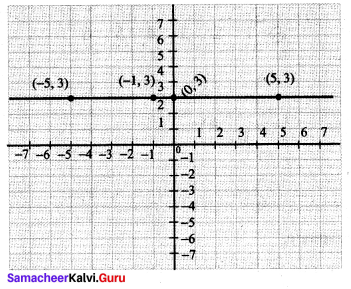When we join the points, we see that they lie on a line which is parallel to x-axis.

(ii)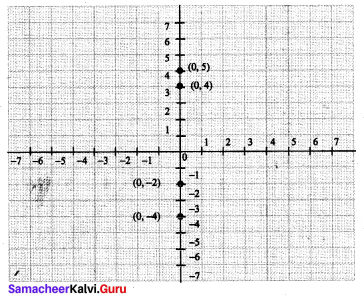When we join the points, we see that they lie on a straight line which is y-axis.

Question 4.
Plot the following points in the coordinate plane. Join them in order. What type of geometrical shape is formed?
(i) (0, 0) (-4, 0) (-4, -4) (0, -4)
(ii) (-3, 3) (2, 3) (-6, -1) (5, -1)
Solution:
(i) (0, 0) (-4, 0) (-4, -4) (0, -4)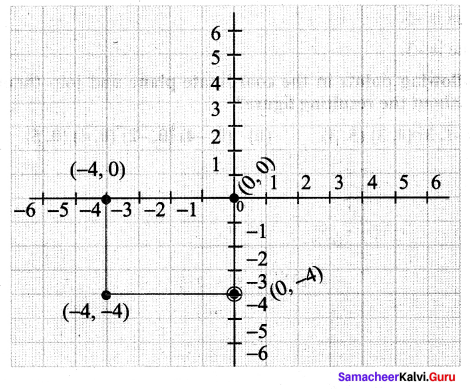The type of geometrical shape is square.

(ii) (-3, 3) (2, 3) (-6, -1) (5, -1)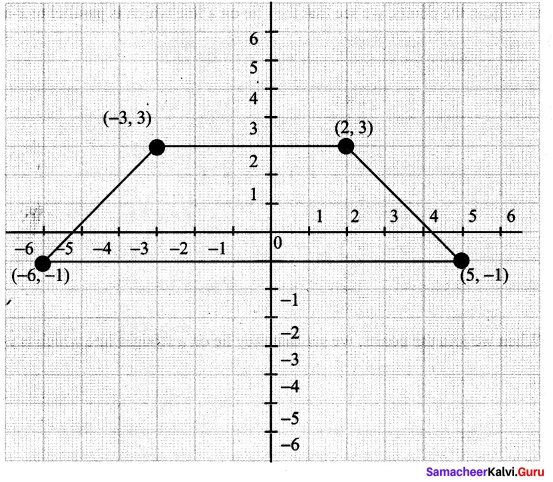The type of geometrical shape is Trapezium.